Dick Cappels' project pages http://www.projects.cappels.org

Return to HOME (More Projects)
A Linear LC Oscillator Component Value to Voltage Converter
Operation of a circuit that displays a voltage as a linear function of inductance or capacitance over a wide range on a voltmeter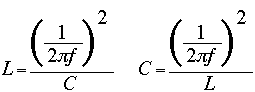Formula 1. Finding unknown inductance or capacitance from resonant frequency,
f and known capacitance C, or known inductance L, respectively.

Overview
A widely used method of determining the values of capacitors and inductors is to connect the unknown device in a resonant circuit with a component of the opposite type with a known value and then the resonant frequency is measured. The values of L and C are then determined from resonant frequency, f, and the other component value by the appropriate arrangement of formula 1. For the sake of simplicity this discussion will focus on measuring inductance, but this technique is applicable to both inductance and capacitance measurement.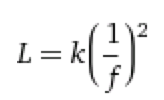Formula 2.Simple relationship of inductance as a function of frequency

Taking  the inductance formula from Formula 1and absorbing the constants into a single variable, k, as shown in Formula 2, the problem of coming up with a linear relationship between f   and L is reduced to thee operations: finding the reciprocal for frequency, square it, then multiply it by a constant k. The constant k can be realized with a gain adjustment somewhere in the circuit, and it will also server as the calibration of the full scale inductance.

A block diagram of nonlinear frequency-to-voltage converter that is the solution to Formula 2 is shown in Figure 1.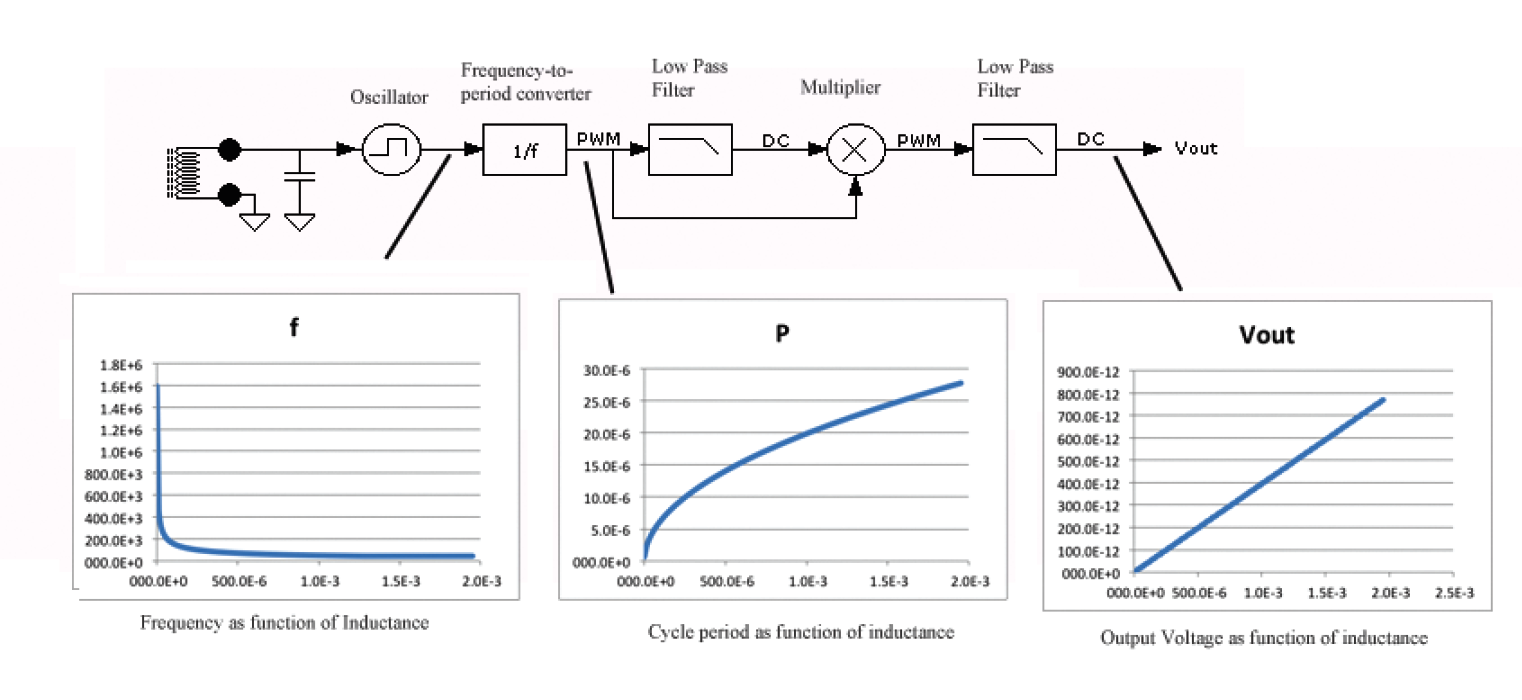Figure 1. An oscillator oscillates at the resonant frequency of a parallel resonant LC circuit. The oscillator frequency is processed to obtain a voltage that is linearly proportional to the inductance or capacitance being measured. The units are arbitrary linear units.

In the system of Figure 1 relies on a simple LC oscillator that, for the purpose of inductance measurement, includes a stable parallel capacitor. The precise value of the capacitor is not critical because adjustment to cover variation from ideal can be done when adjusting K in formula 2, but it should be a temperature stable and slow-aging type of capacitor that does not change much in capacitance over the frequency range of interest. Type COG capacitors are my first choice with type X7R usually being an acceptable substitute.

The oscillation frequency from the LC oscillator is used to generate a pulse with a duty cycle that is linearly proportional to the oscillation period. The period is 1/f in formula 2. All that is left after that is to square the value.

To square the value, thus obtaining the complete function of frequency in formula 2, the duty cycle modulated pulse is averaged in a first order RC low pass filter to produce a voltage proportional to the oscillator period. This voltage if fed into a pulse height-width multiplier along with the same duty cycle modulated pulse to make a voltage proportional to the square of the output of the fist low pass filter. This voltage is then proportional to: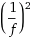, which after adjustment of the scale factor is the function of formula 2.

The Oscillator

Many types of oscillator can be used with this technique. The circuit of Figure 2 is one such circuit and the one used in the demonstration circuit.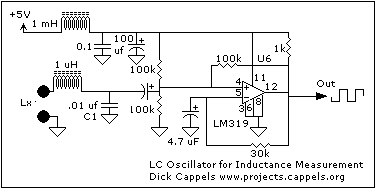Figure 2. The LC Oscillator circuit used in the demonstration.

Some of important considerations in the LC oscillator are that there needs to be a little bit of inductance in the circuit when the inductance under test (Lx in Figure 2) are shorted because the circuit would stop working if the total inductance becomes too small.  This small inductance, shown as 1 uH in figure 2, shows up as a small offset in the output and will be trimmed to nearly zero in the final circuit stage.

The power supply is decoupled to reduce chances for interaction such as cogging between this oscillator and the reference oscillator.

In the case of measuring capacitance
the quality of the resonating inductor is critical. As with the capacitor, the tolerance of the inductance is not very important but the inductance should be stable and its resistance should be low over the frequency range of interest.

Frequency-to-Period
Converter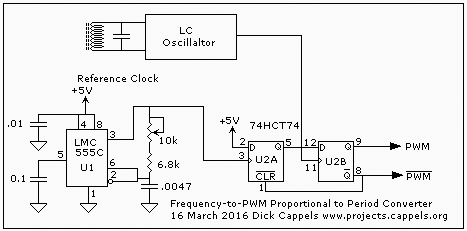Figure
3. U1 sets the duty cycle modulated pulse frequency while interval of the asynchronous LC oscillator sets the width of the pulse.

This circuit block, which is shown in Figure 3, generates pulse width or duty cycle modulation that is the ratio period of the LC oscillator to the period of the reference oscillator. This duty cycle a linear function of 1/f where f is the LC oscillator's frequency.

At rising edge of fixed frequency reference oscillator U5 a logic 1 (5V) into U2A. The rising edge of the clock pulse from the LC oscillator that occurs after the Q output of U2A is set clocks that logic 1 into U2B. The Q output of U2B is the start the pulse portion that is proportional to the LC oscillator's period.  When the Q output of U2B goes high it also resets U2A, making the D input of U2B low so that the next positive edge from the LC oscillator clocks a zero into U2B, ending the portion of the pulse that is proportional to the LC oscillator's period. The circuit begins a new cycle with the arrival of the next positive edge of the reference oscillator U1.

Proper operation requires that the period of the LC oscillator's output must be no more than 50% of the period of the reference oscillator. This is because the the two oscillators are asynchronous. The clocking of the 1 from U2A into U2B can occur at any time in the first 50% of the reference oscillator's period and, in the time remaining after clocking the 1 into U2B, as little as half of the reference clock's period may remain to permit a second rising edge of the pulse from the LC oscillator to clock the 0 into U2B.

F-to-P Over-Range Detection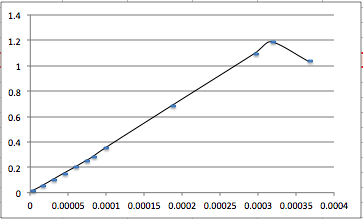Figure 4. This is the converter's output voltage vs inductance measured on an actual prototype. the slope of the function is inverted after the LC oscillator's interval reaches 1/2 the reference oscillator's interval.

As the period of the LC approaches and exceeds that of 1/2 the period of the reference oscillator, the voltage which is the function of average duty cycle begins to have a negative slope with increasing period. This is shown in Figure 4.

Beyond the inflection in Figure 4 the voltage is no longer a voltage linearly related to inductance. It begins to occur when teh rising edge of a reference pulses occur when the Q output of U2B is still high, before being clocked low by the second leading edge of the pulses from the LC oscillator. An additional D latch, U5A can be used to detect the Over-Range condition. In this case, a red LED indicates the undesirable situation, in the case of an inductance meter, that the inductance applied to the circuit is large than the circuit's range permits. This is shown in Figure 5.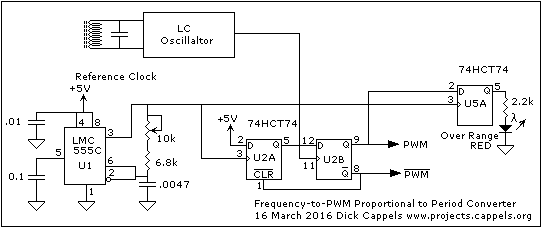Figure 5. D-latch U5A detects the over-range condition.

Low Pass Filters and Multipliers -The Squaring Function

Two cascaded  pulse height-width mulltipliers and their respective low pass filters and buffers perform the function of squaring the signal.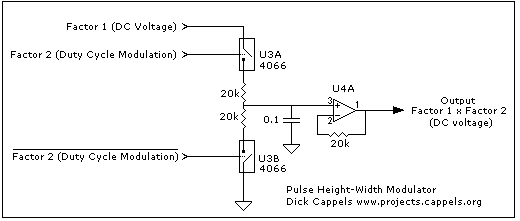Figure 6. Pulse Height-Width Multiplier.

In the pulse height-width modulator a variable amplitude voltage is modulated by a digital signal to produce a variable duty cycle, variable amplitude pulse. When averaged with a suitably long time constant low pass filter this results in a DC voltage that is proportional to the product of an input voltage (Factor 1 in Figure 6) and the duty cycle of the digital signal (Factor 2 in Figure 6), which is in the form of a pair of complimentary duty cycle modulated pulses shown as the PWM signals in Figure 3.

The two 20k resistors reduce the effects of conduction overlap in the two transmission gates might have on the preceding stage and also provide the resistive element in the RC low pass filter.

Since the Frequency-to-Period converter's output pulse width or duty cycle modulation is the ratio of the period of the LC oscillator to the period of the reference oscillator times the reference voltage (here the power supply), the converter's  output signal can be calibrated to remove the effects of component tolerance by adjusting the frequency of the reference oscillator.

The signal swing over the 2.0000 millihenry range is very large since 1 microhenry is represented by 1 microvolt and 2.0000 millihenries is represented by 2.0000 volts

Some important relationships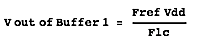Formula
3. Finding the voltage out of the first buffer.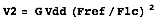Formula 4.Finding the voltage out of the second buffer, which is also the output to the voltmeter.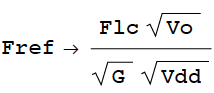Formula 5. Finding the needed reference oscillator frequency for a desired output voltage. Here Vdd which is the power supply voltage, but another stable DC voltage that is  the power supply voltage or lower may be used.

Where:
Fref is the frequency of the reference oscillator,
Vo is the desired output voltage from the second buffer,
Flc is the frequency of the LC oscillator,
G is the gain of the second buffer, and
Vdd is the power supply voltage

Notice that in the special case of the full scale voltage being equal to gain of the output stage (when Vo = G) the formula to find the reference frequency is reduced to (Formula 5.1).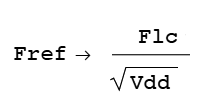Formula 5.1

Example Measured values
Fref = 16.5 kHz
Vo = .060 volts
Flc = 191kHz
G = 2
Vdd = 4.89

In the case of a 5V power supply and a gain of 2x on the second buffer and 2V out at the desired inductance,

Fref = Flc/2.24

Example Circuit Reference Oscillator
In the example circuit a full scale inductance of 2 millihenries was selected, which would be represented by a full scale output voltage of 2,000.0 millivolts so that the inductance can read in microhenries directly from the digital voltmeter.

Here are the conditions:

Resonating with a .01 uf capacitor

Vo is the desired output voltage from the second buffer =2.0000 volts
Flc is the frequency of the LC oscillator = 35.6 kHz
G is the gain of the second buffer = 2
Vdd is the power supply voltage = 5.0V

So for Fref, the reference oscillator was made to be adjustable over a range of frequencies around 15.7 kHz.

It should be clear that the full scale range can be changed by changing the reference frequency and/or the fixed resonating component. Ideally there would be a separate reference oscillator adjustment for each range, even when the fixed resonating component is also switched.

Output Stage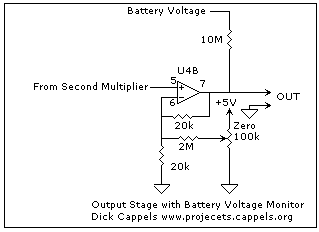Figure 7. The output stage

The output stage is shown in Figure 7. It is merely a nominally X2 amplifier with and offset adjustment and little current injected from the adapter's battery.

There is slight interaction in which the offset voltage has a slight effect on gain, which is the results of design trade-offs resulting from  this being a power and noise sensitive application operating from a single battery.

Care should be taken to use opamps that have a low offset voltage and can swing down to ground. The 2:1 feedback divider can help pull the output voltage into the microvolts region. The amplifier used in the demonstration circuit are contained in a Texas Instruments TLV27L2 dual opamp.

The example circuit is powered by a 9 volt battery. A 10 meg ohm resistor connects the unswitched battery voltage to the output of the circuit. Since the output of the opamp is a high resistance when power to the amplifier is off the approximately 900 nanoamps through the 10 meg resistor causes a voltage drop across the two 20k resistors that make up the feedback divider. The result is that 900 nanoamps x 40k = 36 millivolts appear at the output when the battery is fully charged and when the battery is down to 7 volts which is the maximum dropout voltage of the
78L05ACZ the output voltage will read 28 millivolts. This makes is possible to know when to change the battery without having to open the adapter.

Calibration

In the case of the circuit set up to measure inductance:

Step 1
Center the offset pot.

Step 2
Obtain a reference inductor of known value near full scale and with a reasonably low resistance.  then adjust the reference oscillator frequency to obtain the correct output voltage for the inductor being measured. For example in the case of a 1.70 millihenry reference inductor, adjust the reference frequency oscillator to obtain 1.700 volts on the output of the adapter.

Step 3
Short the measurement terminals and ajust the offset for close to zero volts out. Since there will be a little bit of zero drift and the signal will clip at ground if it tries to go below, I accept a few hundred microvolts of offset. If I am measuring in the microhenry range I mentally deduct the fractions of a microhenry offset
.

Step 4
Remove the short and measure the reference inductor. If the reading is too far off, repeat steps 2 through 4 until both the zero and full scale readings are satisfactory.

Complete Schematic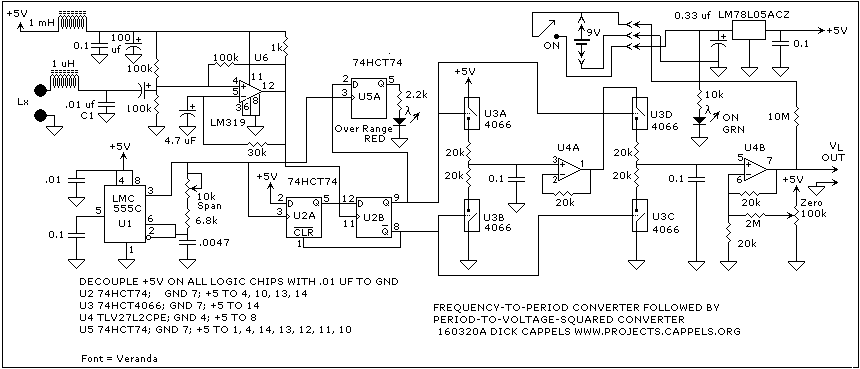Figure 8. The complete schematic of the adapter. The output signals leave the case via captive leads that plug into a digital voltmeter.

Find updates at www.projects.cappels.org

HOME (More Projects)

Contents ©20016 Richard Cappels All Rights Reserved. Find updates at www.projects.cappels.org

First published in March, 2016.

You can send  email to me at projects(at)cappels.org. Replace "(at)" with "@" before mailing.
Use of information presented on this page is for personal, nonprofit educational and noncommercial use only. This material (including object files) is copyrighted by Richard Cappels and may not be republished or used directly for commercial purposes. For commercial license, click below.Liability Disclaimer and intellectual property notice:

(Summary: No warranties, use these pages at your own risk. You may use the information provided here for personal and educational purposes but you may not republish or use this information for any commercial purpose without explicit permission.) I neither express nor imply any warranty for the quality, fitness for any particular purpose or  user, or freedom from patents or other restrictions on the rights of use of any software, firmware, hardware, design, service,information, or advice provided, mentioned,or made reference to in these pages. By utilizing or relying on software, firmware, hardware, design, service,information, or advice provided, mentioned, or made reference to in these pages, the user takes responsibility to assume all risk and associated with said activity and hold Richard Cappels harmless in the event of any loss or expense associated with said activity. The contents of this web site, unless otherwise noted, is copyrighted by Richard Cappels. Use of information presented on this site for personal, nonprofit educational and noncommercial use is encouraged, but unless explicitly stated with respect to particular material, the material itself may not be republished or used directly for commercial purposes. For the purposes of this notice, copying binary data resulting from program files, including assembly source code and object (hex) files into semiconductor memories for personal, nonprofit educational or other noncommercial use is not considered republishing. Entities desiring to use any material published in these  pages for commercial purposes should contact the respective copyright holder(s).